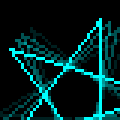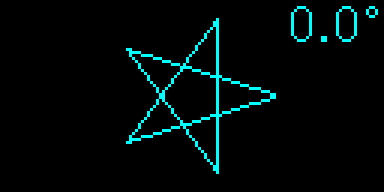Yoctopuce displays and double buffering

# Yoctopuce displays and double bufferingWe recently noticed that, when drawing on a raster display is involved, not everybody thinks about "double buffering" as the go to technique. That's why, this week, we are proposing a short article explaining how this technique works and why it is so easy to use it with Yoctopuce displays.

## Raster displays and their problems

Raster displays are the ones based on a pixels grid, they are the most common. When one has to display an ever changing information on such displays, no matter if it's text or graphics, one almost always works the same way:

1. Clear the old display contents
2. Re-draw the new display contents
3. Wait for small amount of time if needed
4. Do it again

As long as the time required the build the display contents is much shorter than the time for the electronics to refresh the physical display, everything is fine. However, the more complex and time-consuming the construction of the display contents is, the more likely it is that the screen will be refreshed before the contents construction is finished. In such situations, the result is usually a more or less incomplete image flickering on the display.

Here is a small example, written in Python, showing a rotating star on a Yoctopuce display:

import sys, math
from yocto_api import *
from yocto_display import *

# setup the Yoctopuce API
errmsg = YRefParam()
if YAPI.RegisterHub("usb", errmsg) != YAPI.SUCCESS:
sys.exit("init error" + errmsg.value)

# Finds the Display
disp = YDisplay.FirstDisplay()
if disp is None: sys.exit("No display found")

# display clean up
disp.resetAll()

# retreive the display size
w = disp.get_displayWidth()
h = disp.get_displayHeight()

# retreive the first layer
l1 = disp.get_displayLayer(1)
l1.selectFont("Medium.yfm")

# some constants
spikeCount = 5
delta      = 2*math.pi / spikeCount
radius     = (h/2)  -5
angle      = 0

while True:
l1.clear()
l1.drawText(w,0,YDisplayLayer.ALIGN.TOP_RIGHT,"%.1f"%(180*angle/math.pi)+"°")
l1.moveTo( w/2 + radius*math.cos(angle), h/2 + radius*math.sin(angle))
for i in range( 1,spikeCount+1):
a=angle+(2*i)*delta
l1.lineTo( w/2 + radius*math.cos(a), h/2 + radius*math.sin(a))
angle =angle+ delta /10
if angle>=2*math.pi :  angle=  angle-2*math.pi;
YAPI.Sleep(40,errmsg)

The result is a quite unpleasant flickering image. Here is an animation based on frames grabbed from a Yocto-MaxiDisplay running the above program.Without double buffering

## The solution: double buffering

The technique to avoid such flickering is to construct the image to be displayed in a memory buffer rather than directly in the video memory. Once the image is ready, the contents raster data are transferred from the construction buffer to the video memory. Hence the "double buffering" term. Since this is just a matter of copying a memory block from one buffer to another, this is a a very quick operation.

Usually this technique requires graphic primitives that are able to work in any arbitrary memory buffer instead of working directly with the video memory, but Yoctopuce display are specials.

### The API for Yoctopuce displays

When one calls a drawing primitive with the Yoctopuce API, one doesn't write directly in the video memory but in a buffer which behave like a virtual layer. Meanwhile, the display's electronics constantly compute a layers merge which is displayed on the actual screen. This is very similar to the layer system found in any modern drawing software. Actually,

• Each of these layers can be set as invisible
• One can draw in any invisible layer
• Two layers contents can be swapped, no matter their visibility state

We can therefore implement the double buffering technique by choosing two layers, one of which will be invisible. Then we just have to draw in the invisible layer, and once the drawing operation is finished, we just swap the contents of the two layers. In practice, this is just a matter of three more lines added to the previous example:

....
# retreive the first layer
l1 = disp.get_displayLayer(1)
l1.selectFont("Medium.yfm")
l1.hide()                                      #DOUBLE BUFFERING EXTRA LINE 1
l2 = disp.get_displayLayer(2)                  #DOUBLE BUFFERING EXTRA LINE 2

# some constants
spikeCount = 5
delta      = 2*math.pi / spikeCount
radius     = (h/2)  -5
angle      = 0

while True:
l1.clear()
l1.drawText(w,0,YDisplayLayer.ALIGN.TOP_RIGHT,"%.1f"%(180*angle/math.pi)+"°")
l1.moveTo( w/2 + radius*math.cos(angle), h/2 + radius*math.sin(angle))
for i in range( 1,spikeCount+1):
a=angle+(2*i)*delta
l1.lineTo( w/2 + radius*math.cos(a), h/2 + radius*math.sin(a))
disp.swapLayerContent(1,2)                   #DOUBLE BUFFERING EXTRA LINE 3
angle =angle+ delta /10
if angle>=2*math.pi :  angle=  angle-2*math.pi;
YAPI.Sleep(40,errmsg)

And here is the result:With double buffering

## Conclusion

The result is indisputable, with Yoctopuce displays, double buffering is so easy to implement, that no one should do without it. Finally, note that in of the case of the Yoctopuce API, drawing in an invisible layer is usually faster than drawing in a visible one because commands sent to invisible layers are buffered while those sent to visible layers are sent on by one in real time.

 Add a comment No comment yet Back to blog